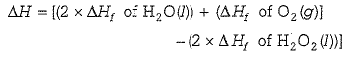# NEETprep Bank NEET Chemistry Thermodynamics Questions Solved

Work done in reversible adiabatic process is given by:

(a) 2.303RTlog(V2/V1)                      (b)

(c) 2.303RTlog(V2/V1)                      (d) none of these

(b) This is the derived formula for Wrev in adiabatic process.

Difficulty Level:

• 29%
• 42%
• 17%
• 13%

Change in enthalpy for reaction,

2H2O2(l)$\to$ 2H2O(l) + O2(g)

if the heat of formation of H2O2(l) and H2O(l) are -188 and -286 kJ/mol respectively is

(a) -196 kJ/mol              (b) + 196 kJ/mol

(c) +948 kJ/mol             (d) -948 kJ/mol

(a) 2H2O2(l) $\to$2H2O(l) + O2(g) $∆$H=?= [(2x-286) + (0) -(2 x -188)]

=[-572 + 376] = -196 kJ/mol

Difficulty Level:

• 51%
• 28%
• 15%
• 8%

One mole of a non-ideal gas undergoes a change of state (2.0 atm, 3.0 L, 95 K)$\to$(4.0 atm, 5.0 L, 245 K) with a change in internal energy, $∆$U = 30.0 L atm. The change in enthalpy ($∆$H) of the process in L atm is:

(a) 40.0

(b) 42.3

(c) 44.0

(d) not defined, because pressure is not constant

(c) H=U + PV

... H2-H1 = U2-U1+(P2V2 - P1V1)

...     $∆$H = 30 + (4x5 - 2x3) = 44 L atm

Difficulty Level:

• 14%
• 26%
• 32%
• 30%

At absolute zero, the entropy of a perfect crystal is zero. This is........... of thermodynamics.

(a) first law               (b) second law

(c) third law              (d) none of these

(c) This is definition of third law of thermodynamics.

Difficulty Level:

• 16%
• 35%
• 45%
• 5%

At constant pressure and temperature, the direction of any chemical reaction is one where, the...... decrease.

(a) entropy                (b) enthalpy

(c) Gibbs energy        (d) none of these

(c) Spontaneous process shows a decrease in $∆$G

Difficulty Level:

• 23%
• 29%
• 44%
• 6%

The work done by a mass less piston in causing an expansion $∆$V (at constant temperature), when the opposing pressure, P is variable, is given by:

(a) W= $-\int \mathrm{P}∆\mathrm{V}$                (b) W=0

(c) W= -P$∆$V                      (d) none of these

(a) Wrev; note that opposing pressure is not constant throughout.

Difficulty Level:

• 59%
• 12%
• 27%
• 3%

Entropy decreases during:

(a) crystallization of sucrose from solution

(b) rusting of iron

(c) melting of ice

(d) vaporization of camphor

(a) During solidification disorder decreases.

Difficulty Level:

• 59%
• 13%
• 18%
• 11%

Entropy change of vaporisation at constant pressure is given by:

(1) This is derived formula.

Difficulty Level:

• 60%
• 14%
• 21%
• 7%

The entropy change for the reaction given below,

2H2 (g) + O2 (g) $\to$2H2O(l)

is ... at 300 K. Standard entropies of H2 (g), O2(g) and H2O(l) are 126.6, 201.20 and 68.0 JK-1mol-1 respectively.

(a) -318.4 JK-1mol-1                           (b) 318.4 JK-1mol-1

(c) 31.84 JK-1mol-1                             (d) none of these

(a)

= 2 x 68-[2 x 126.6 + 201.20]

= -318.4 JK-1mol-1

Difficulty Level:

• 59%
• 25%
• 11%
• 8%

If S$°$ for H2, Cl2 and HCl are 0.13, 0.22 and 0.19 kJ K-1mol-1 respectively. The total change in standard entropy for the reaction, H2 + Cl2 $\to$2HCl is:

(a) 30 J K-1mol-1                         (b) 40 J K-1mol-1

(c) 60 J K-1mol-1                         (d) 20 J K-1mol-1

(a) $∆\mathrm{S}$=SP-SR

=(2 x0.19) - 0.13-0.22

= 0.03 kJ K-1mol-1

= 30 JK-1mol-1

Difficulty Level:

• 67%
• 16%
• 12%
• 7%